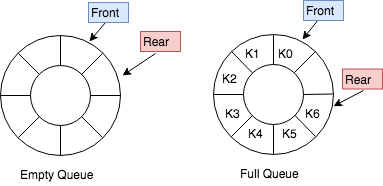# 栈与队列

## 栈

### 栈的实现方式

• 顺序栈（Array-based Stack）
• 使用向量实现，本质上是顺序表的简化版
• 向量尾部可作为栈顶
• 使用单链表方式存储
• 其中指针的方向是从栈顶向下链接
``````  // 入栈操作的链式实现
bool lnksStack<T>:: push(const T item) {
top = tmp;
size++;
return true;
}
data = info;
next = nextValue;
}
``````
• 顺序栈和链式栈的比较
• 时间效率
• 所有操作都只需要常数时间
• 顺序栈和链式栈在时间效率上难分伯仲
• 空间效率
• 顺序栈需要一个固定长度
• 链式栈长度可变，但增加结构开销
• 实际应用中，顺序栈比链式栈用的更广泛
• 顺序栈容易根据栈顶位置，进行相对位移，快速定位并读取栈内部的元素
• 顺序栈读取内部元素时间为`O(1)`,链式栈需要沿着栈顶指针游走，显然慢些，读取第k个元素需要的时间为`O(k)`
• 一般来说，栈不允许“读取内部元素”，只能在栈顶操作

### 计算表达式的值

• 表达式的递归定义
• 基本符号集合：`{0，1，2，3，... ，9，+，-，*，/,(,)}`
• 语法成分集合: `{<表达式>,<项>,<因子>,<常数>,<数字>}`
• 中缀表达式 `23+(34*45)/(5+6+7)`
• 运算符在中间，需要括号改变优先级
• 中缀表达式求值： 二叉树的中序遍历
• 语法公式（巴克斯范式）：
• 后缀表达式 `23 34 45 * 56 + 7 + / +`
• 又称逆波兰表达式
• 运算符在后面，不需要括号
• 后缀表达式求值
• 二叉树的后序遍历
• 使用栈
• 当遇到一个操作数，入栈
• 当遇到一个运算符，从栈中两次取出栈顶，按照运算符对这两个操作数进行计算，然后将结果入栈

## 队列

• 先进先出
• 限制访问点的线性表
• 按照到达的顺序来释放元素
• 所有的插入在表的一端进行，所有的删除在表的另一端进行
• 主要元素
• 队头-front
• 队尾-rear
• 抽象数据类型
``````template <class T>
class Queue {
public: // 队列的运算集
void clear(); // 变为空队列
bool enQueue(const T item);// 将item插入队尾，成功则返回真，否则返回假
bool deQueue(T & item) ;// 返回队头元素并将其从队列中删除，成功则返回真
bool getFront(T & item); // 返回队头元素，但不删除，成功则返回真
bool isEmpty(); // 返回真，若队列已空
bool isFull(); // 返回真，若队列已满
};
``````

### 实现方式

• 顺序队列
• 使用线性表做环形表示，空间提前分配好
• 维护`front``rear`做队头，队尾的游标
• 空队列`rear``front`前面
• 插入删除时间复杂度为`O(1)```````template <class Elem>
class Aqueue : public Queue<Elem> {
private:
int size; // 队列的最大容量
int front; // 队首元素指针
int rear; // 队尾元素指针
Elem *listArray; // 存储元素的数组
public:
AQueue(int sz=DefaultListSize) {// 让存储元素的数组多预留一个空位
size = sz+1; // size数组长，sz队列最大长度
rear = 0; front = 1; // 也可以rear=-1; front=0
listArray = new Elem[size];
}
~AQueue() { delete [] listArray; }
void clear() { front = rear+1; }
int length() { reutrn (rear + 1 -front)%size; }
``````
• 入队
• 在队尾插入，移动`rear`指针
``````  bool enqueue(const Elem& it){
if(((rear+2)%size) == front){
return false;
}else{
rear = (rear+1)%size;
listArray[rear] = it;
return true;
}
}
``````
• 出队
• 依靠移动`front`指针，不进行delete元素的操作
``````  bool dequeue(Elem& it){
if(length() == 0 ){
return false;
}
it = listArray[front];
front = (front+1)%size;
return true;
}
``````
• 链式队列
• 用单链表方式存储，队列每个元素对于链表中的一个节点
• 插入时间复杂度为`O(1)`

### 顺序队列和链式队列比较

• 顺序队列
• 固定存储空间
• 链式队列
• 可以满足大小无法估计的情况
• 都不允许访问队列内部元素

• 环形队列
• 线性表在部分元素出队后会造成空间的浪费，解决这个问题，引入环形队列，它是一个首尾相连的FIFO的数据结构，采用数组的线性空间,数据组织简单。能很快知道队列是否满为空。
• 插入时间复杂度为`O(1)`

## 队列与栈的经典问题

### 表达式求值

1. 如果当前是数字，向后遍历直到遇到符号，输出数字
2. 如果当前是`(`，直接入栈
3. 如果当前是`)`，弹出栈中所有符号并输出，直到遇到`(`，弹出`(`
4. 如果当前是`+,-,*,/`，根据优先级入栈
• 如果当前符号优先级>栈顶元素，直接入栈
• 如果当前符号优先级<=栈顶个元素，弹出栈顶元素，直到遇到`(`或者优先级更高的元素
``````vector<string> infix2postfix(string& postfix){
vector<string> postfix;
stack<char> stk;
int i = 0;
string num = "";
while(i<postfix.size()){
char c = postfix[i];
if( isspace(c) ){
i++;
continue;
}else if( isdigit(c) ){
do{
num+=c;
i++;
c = char[i];
}while(isdigit(c));
postfix.push_back(num);
num = "";
continue;
}else if( c == '('){
stk.push_back(c);
}else if( c== ')' ){
while(!stk.empty() && stk.top()!='('){
postfix.push_back(string(1,stk.top()));
stk.pop();
}
//pop ')'
stk.pop();
}else if( isoperator(c) ){
while(!stk.empty() && level(c) <= level(stk.top())){
postfix.push_back(string(1,stk.top()));
stk.pop();
}
stk.push(c);
}
i++
}
//输出栈中符号
while(!stk.emtpy()){
postfix.push_back(string(1,stk.top()));
stk.pop();
}
return postfix;
}
``````

``````int calculate(string& infix){
vector<string> postfix = infix2postfix(infix);
stack<long> stk;
int sum=0;
for(auto &s : postfix){
if(isoperator(s)){
long x = stk.top();
stk.pop();
long y = stk.top();
stk.pop();
if(s == "+"){
stk.push(x+y);
}else if(s =="-"){
stk.push(y-x);
}else if(s =="*"){
stk.push(x*y);
}else if(s == "/"){
stk.push(y/x);
}
}else{
stk.push(stol(s));
}
}
int sum = 0;
while(!stk.empty()){
sum += stk.top();
stk.pop();
}
return sum;
}
``````